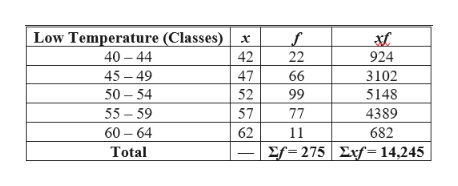# Find the mean of the data summarized in the given frequency distribution. Compare the computed mean to the actual mean of51.951.9degrees. Low Temperature​(circle◦​F)40minus−4445minus−4950minus−5455minus−5960minus−64 Frequency2266997711 The mean of the frequency distribution isnothingdegrees.

Question
285 views
Find the mean of the data summarized in the given frequency distribution. Compare the computed mean to the actual mean of
51.951.9
degrees.

 Low Temperature ​(circle◦​F) 40minus−44 45minus−49 50minus−54 55minus−59 60minus−64 Frequency 22 66 99 77 11
The mean of the frequency distribution is
nothing
degrees.
check_circle

Step 1

Note:

Hi! Thank you for posting the question. However, in our display of the website, the actual mean is showing as “51.951.9”. We have considered it as 51.9 and proceeded with the problem. Also, the classes of Low Temperature are showing as “40minus–44”, “45minus–49”, etc. We have considered these as 40 – 44, 45 – 49, etc., as in “40 to 44”, “45 to 49”,… and solved the problem.

Step 2

Calculation:

In order to find the mean of a grouped frequency, the mid-point of each class is considered as the representative of that class.

If the mid-point of a class is taken as x and the frequency as f, then the mean is: (Σxf) / (Σf).

The following table shows the calculation of the mean:help_outlineImage TranscriptioncloseLow Temperature (Classes)x 40 44 42 22 924 45-49 47 66 3102 5148 4389 50 54 55 59 52 99 57 77 60-64 62 11 682 Σf-275|Σf-14,245 Total fullscreen
Step 3

The mean and its comparison:

Thus, the mean of the grouped frequency distribution is:

mean = (Σxf) / (Σf)

= 14,245/275

= 51.8.

The actual mean is 51.9.

The mean of the grouped frequency distribution (51.8°F) and the actua...

### Want to see the full answer?

See Solution

#### Want to see this answer and more?

Solutions are written by subject experts who are available 24/7. Questions are typically answered within 1 hour.*

See Solution
*Response times may vary by subject and question.
Tagged in

### Data